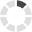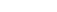### Site InformationLoading... Please wait...

# Ashworth MA260 Exam 4 Answers / Statistical Analysis I

\$14.99

## Product Description

MA260 Statistical Analysis Online Exam 4 Answers (Ashworth College)

Question 1 of 20

5.0/ 5.0 Points

If light bulbs have lives that are normally distributed with a mean of 2500 hours and a standard deviation of 500 hours, what percentage of light bulbs have a life less than 2500 hours?

Question 2 of 20

5.0/ 5.0 Points

Decide which of the described variables likely have a normal or near-normal distribution.

a) The amount of change held by a teacher at the end of each day for a year

b) The amount of pocket money held by each student at a mid-sized liberal arts college at a given time

c) The number of heads that show when two coins are tossed

A. a               B. b           C. c                    D. None

Question 3 of 20

5.0/ 5.0 Points

The scores on a certain test are normally distributed with a mean score of 58 and a standard deviation of 4. What is the likelihood that a sample of 90 students will have a mean score of at least 58.4216?

A. 0.8413        B. 0.3413            C. 0.3174        D. 0.1587

Question 4 of 20

5.0/ 5.0 Points

The lifetimes of light bulbs of a particular type are normally distributed with a mean of 270 hours and a standard deviation of 11 hours. What percentage of the bulbs has lifetimes that lie within 2 standard deviations of the mean on either side?

Apply the 68-95-99.7 rule to this questions.

A. 68% B. 1005 C. 99.7% D. 95%

Question 5 of 20

5.0/ 5.0 Points

The amount of Jen’s monthly electric bill is normally distributed with a mean of \$160 and a standard deviation of \$14. Fill in the blanks.

95% of her electric bills are between __________ and __________.

Apply the 68-95-99.7 rule to this question.

A. \$140, \$190      B. \$132, \$190      C. \$140, \$188     D. \$132, \$188

Question 6 of 20

5.0/ 5.0 Points

The volumes of soda in quart soda bottles are normally distributed with a mean of 32.3 oz and a standard deviation of 1.2 oz. What is the probability that the volume of soda in a randomly selected bottle will be less than 32 oz?

A. 0.5012    B. 0.5010        C. 0.4015         D. 0.4013

Question 7 of 20

5.0/ 5.0 Points

The lifetimes of projector bulbs of a particular type are normally distributed with a mean of 470 hours and a standard deviation of 15 hours. What percentage of the bulbs has lifetimes that lie within 2 standard deviations of the mean on either side?

Apply the 68-95-99.7 rule to this question.

A. 68% B. 99.7% C. 95% D. 100%

Question 8 of 20

5.0/ 5.0 Points

The mean score on the exit examination for an urban high school is 63 with a standard deviation of 9. What is the standard deviation of the distribution of sample means with a sample size of 9?

A. 2 B. 3 C. 4 D. 4.1

Question 9 of 20

5.0/ 5.0 Points

The amount of Jen’s monthly phone bill is normally distributed with a mean of \$50 and a standard deviation of \$10. Find the 25th percentile.

Question 10 of 20

5.0/ 5.0 Points

If light bulbs have lives that are normally distributed with a mean of 2500 hours and a standard deviation of 500 hours, approximately what percentage of light bulbs has a life of more than 3000 hours?

Question 11 of 20

5.0/ 5.0 Points

A bank’s loan officer rates applicants for credit. The ratings are normally distributed with a mean of 200 and a standard deviation of 50. If an applicant is randomly selected, find the probability of a rating that is between 225 and 275.

A. 0.2416 B. 0.2418 C. 0.2417 D. 0.2420

Question 12 of 20

5.0/ 5.0 Points

The systolic blood pressure of 18-year-old women is normally distributed with a mean of 120 mm Hg and a standard deviation of 12 mm Hg. What percentage of 18-year-old women have a systolic blood pressure that is within 3 standard deviations of the mean on either side?

Apply the 68-95-99.7 rule to this question.

A. 68% B. 95% C. 100% D. 99.7%

Question 13 of 20

5.0/ 5.0 Points

Which of the following statements concerning areas under the standard normal curve is/are true?

a) If a z-score is negative, the area to its right is greater than 0.5.

b) If the area to the right of a z-score is less than 0.5, the z-score is negative.

c) If a z-score is positive, the area to its left is less than 0.5.

A. a, c      B. b, c     C. a, b     D. a

Question 14 of 20

5.0/ 5.0 Points

The systolic blood pressures of the patients at a hospital are normally distributed with a mean of 136 mm Hg and a standard deviation of 12 mm Hg. Find the two blood pressures having these properties: the mean is midway between them and 76.98% of all blood pressures are between them.

A. 121.6, 152.4 B. 121.6, 150.4 C. 123.6, 150.4 D. 122.6, 148.4

Question 15 of 20

0.0/ 5.0 Points

Decide which of the described variables likely have a normal or near-normal distribution.

1. The number of credits remaining until graduation for the students in a small liberal arts college

2. The heights of male students in an advanced placement mathematics class

3. The number of sixes showing when two dice are rolled

A. a B. b C. c D. None

Question 16 of 20

5.0/ 5.0 Points

The annual precipitation for one city is normally distributed with a mean of 72 inches and a standard deviation of 3.5 inches. Fill in the blanks.

In 99.7% of the years, the precipitation in this city is between __________ and __________ inches.

Apply the 68-95-99.7 rule to this questions.

A. 61.5, 82. 5 B. 82.5, 61.5 C. 61.5, 85.5 D. 63.5, 82.5

Question 17 of 20

0.0/ 5.0 Points

The area under the standard normal curve between 1 and 2 is equal to 0.1359. Scores on a particular aptitude test are normally distributed with a mean of 100 and a standard deviation of 10. Which of the following are equal to 13.59%?

a) The percentage of scores between 120 and 130

b) The percentage of scores between 110 and 120

c) The percentage of scores between 80 and 90

d) The percentage of scores between 90 and 120

A. b          B. b, c           C. d          D. a, b

Question 18 of 20

5.0/ 5.0 Points

The diameters of bolts produced by a certain machine are normally distributed with a mean of 0.25 inches and a standard deviation of 0.01 inches. What percentage of bolts will have a diameter greater than 0.24 inches?

A. 79.13% B. 82.46% C. 84.13% D. 84.46%

Question 19 of 20

0.0/ 5.0 Points

Which of the following statements concerning the standard normal curve is/are true (if any)?

a) The area under the standard normal curve to the left of  -3 is zero.

b) The area under the standard normal curve between any two z-scores is greater than zero.

c) The area under the standard normal curve between two z-scores will be negative if both z-scores are negative.

d) The area under the standard normal curve to the left of any z-score is less than 1.

Question 20 of 20

5.0/ 5.0 Points

A final exam in Math 160 has a mean of 73 with standard deviation 7.73. Assume that a random sample of 24 students is selected and the mean test score of the sample is computed. What percentage of sample means are less than 70?

A. 19.46%

B. 12.85%

C. 2.87%

D. 13.46%

## Customers also viewed

• \$14.99• \$14.99• \$14.99• \$24.99• \$24.99## Related Products

Click the button below to add the Ashworth MA260 Exam 4 Answers / Statistical Analysis I to your wish list.